Points to Remember- Exponents and Powers

# Points to Remember- Exponents and Powers Notes | Study Mathematics (Maths) Class 8 - Class 8

## Document Description: Points to Remember- Exponents and Powers for Class 8 2022 is part of Mathematics (Maths) Class 8 preparation. The notes and questions for Points to Remember- Exponents and Powers have been prepared according to the Class 8 exam syllabus. Information about Points to Remember- Exponents and Powers covers topics like and Points to Remember- Exponents and Powers Example, for Class 8 2022 Exam. Find important definitions, questions, notes, meanings, examples, exercises and tests below for Points to Remember- Exponents and Powers.

Introduction of Points to Remember- Exponents and Powers in English is available as part of our Mathematics (Maths) Class 8 for Class 8 & Points to Remember- Exponents and Powers in Hindi for Mathematics (Maths) Class 8 course. Download more important topics related with notes, lectures and mock test series for Class 8 Exam by signing up for free. Class 8: Points to Remember- Exponents and Powers Notes | Study Mathematics (Maths) Class 8 - Class 8
 1 Crore+ students have signed up on EduRev. Have you?

Points to Remember
• The numbers with negative exponents also obey the following laws:
(i) xm * xn = xm+n
(ii) xm ÷ xn = xm–n
(iii) xm * bm = (xb)m
(iv) x0 = 1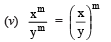• A number is said to be in the standard form, if it is expressed as the product of a number between 1 and 10 and the integral power of 10.
• Very small numbers can be expressed in standard form using negative exponents.

WE KNOW THAT
When we write 54, it means 5 * 5 * 5 * 5, i.e. 5 is multiplied 4 times. So 5 is the base and 4 is the exponent. We read 54 as “5 raised to the power 4’’.
We also know the following laws of exponents
(i) xm ⋅ xn = xm+n
(ii) xm ÷ xn = xm–n
(iii) (xm)n = xm*n
(iv) xm * ym = (xy)m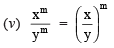The value of any number raised to 0 is 1, i.e. a0 = 1.
We express very small or very large numbers in standard form (i.e scientific notation) for
Example: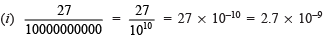(ii) 3600000000000 = 36 * 1011 = 3.6 * 1012
So, 3.6 * 1012 is the standard form.

POWER WITH NEGATIVE EXPONENTS
For a non-zero integer x, we have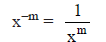or  x–m * xm = 1
So x–m is the reciprocal (or the multiplicative inverse) of xm and vice versa.
For example: (i) Reciprocal of 8–7 = 87 and
(ii) Reciprocal of 8= 8–7

Solved Examples
Ques 1: Find the multiplicative inverse of the following.
(i) 2–4
(ii) 10–5
(iii) 7–2
(iv) 5–3
(v) 10–100
Solution:

(i) The multiplicative inverse of 2–4 is 24.
(ii) The multiplicative inverse of 10–5 is 105
(iii) The multiplicative inverse of 7–2 is 72.
(iv) The multiplicative inverse of 5–3 is 53.
(v) The multiplicative inverse of 10–100 is 10100.

Ques 2: Find the value of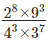Solution:
By using rule 2 and 3 –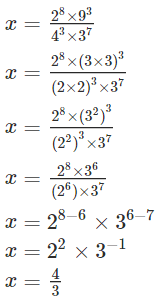Ques 3: Solve the following: (-3)2 × (5/3)3
Solution:
(-3)2 × (5/3)3
= (-3 × -3) × ( ( 5 × 5 × 5 ) / ( 3 × 3 × 3 ) )
= 9 × (125/27)
= (125/3)

Ques 4: If x11 = y0 and x=2y, then y is equal to
a. 1/2
b. 1
c. -1
d. -2
Solution:
Option A. x11 = y0 => x11 = 1 => x = 1. Given, x = 2y hence, y = x/2 =1/2

Ques 5: By what number (4)-3 be multiplied so that the product become 1/16?
Solution:
4 Simplest way to to solve this would be:
1/16 = 1/42 = (4)-2
(4)-3 × 4 = (4)-2

Ques 6: What is the value of 63 ?
a. 18
b. 216
c. 729
d. 1296

Solution: Option b.

63
= 6 × 6 × 6
= 36 × 6
= 216

Ques 7: What is the value of (-2)-5?
a. -0.03125
b. 0.03125
c. 10
d. 32

Solution: Option A.
(-2)-5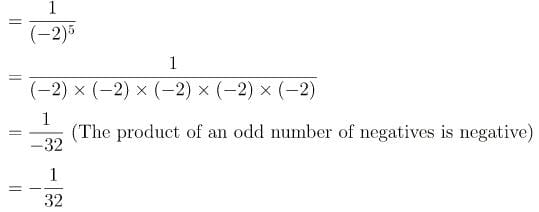= -0.03125

The document Points to Remember- Exponents and Powers Notes | Study Mathematics (Maths) Class 8 - Class 8 is a part of the Class 8 Course Mathematics (Maths) Class 8.
All you need of Class 8 at this link: Class 8

## Mathematics (Maths) Class 8

187 videos|323 docs|48 tests
 Use Code STAYHOME200 and get INR 200 additional OFF

## Mathematics (Maths) Class 8

187 videos|323 docs|48 tests

Track your progress, build streaks, highlight & save important lessons and more!

,

,

,

,

,

,

,

,

,

,

,

,

,

,

,

,

,

,

,

,

,

;